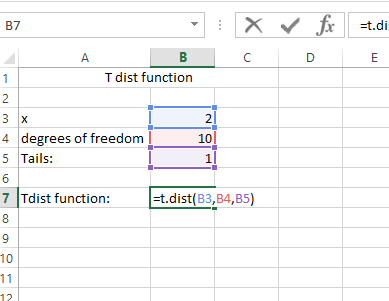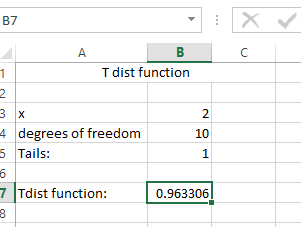#### How to calculate t value in Excel

In this Excel tutorial lesson, we will learn a very useful financial term known as T-Value.

## Understanding T-Value

A T-Value is a statistical calculation using the mean, standard deviation, and degrees of freedom. It will help in financial projections and forecasting.

With this information, a business owner can calculate the statistical low and high for earnings in a specific month and with a degree of confidence that the future earnings will fall within that range, which helps with better business planning. Projections might suggest that the business can expect to gross \$150,000 in May and the T-Value might tell them that number has a 90-percent rate of confidence. If the confidence rate is less than 50-percent, the business can plan for the potential of a lower return and work to increase the T-Value for future months and years.

## The syntax

Let us show you how to calculate the T value using a simple formula in Excel:

The Formula =T.DIST(x,deg_freedom,tails)

The T.DIST function uses the following arguments:

• X (required argument) – This is the numeric value at which we wish to evaluate the T Distribution.
• Deg_freedom (required argument) – An integer that indicates the number of degrees of freedom.
• Tails (required argument) – This specifies the number of distribution tails that would be returned. If tails =1, T.DIST returns the one-tailed distribution. If tails = 2, T.DIST returns the two-tailed distribution.

## Data

Let’s create the data for the calculation:

T dist function

• x: 2
• degrees of freedom: 10
• Tails: 1

## The T.DIST formula

The formula is applied as below:

=T.DIST(B3,B4,B5)Result is:The Tdist function result is 0.963306

## The T.INV formula

To calculate the t-value in Microsoft Excel, you can also use the “T.INV” function. The T.INV function calculates the one-tailed probability of the t-distribution. The function has two arguments:

1. Probability (p): The probability associated with the t-distribution. This argument must be between 0 and 1, exclusive.
2. Degrees of freedom (df): The number of degrees of freedom in the t-distribution. This argument must be greater than 0.

Example:

To calculate the t-value with a probability of 0.025 and degrees of freedom of 10, you would enter the following formula in a cell in Excel:

=T.INV(0.025, 10)

This formula returns the critical T-value for a one-tailed distribution with a 2.5% significance level and 10 degrees of freedom.

This would return the t-value of -1.83311293.

## Interpreting T-Values

• A high T-value indicates that the observed difference is statistically significant, implying that the estimate or prediction is reliable.
• A low T-value suggests that the observed difference is not statistically significant, meaning that the estimate may not be reliable.
• The choice of one-tailed or two-tailed distribution depends on the specific hypothesis or research question.

In finance, T-values are valuable for assessing the confidence and reliability of financial projections, risk assessments, and investment decisions. By understanding how to calculate and interpret T-values, financial analysts can make more informed choices based on statistical evidence.